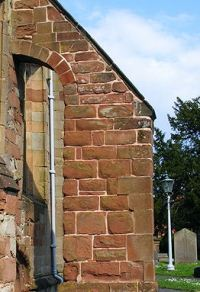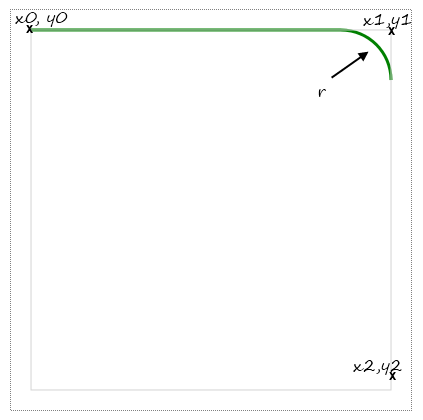# Drawing an arc in Canvas with the arcTo methodFlyings buttress has the arcTo shape

The arcTo method defines an arc in the extension of a straight line or another figure.

The difference with the arc method is that the point of departure and arrival are not defined by an angle but by coordinates.

In fact the arcTo plot has nothing intuitive, the coordinate points are guidelines rather than exact locations, and the same figure can be obtained with different coordinates for the end point. The figure above may allow a better understanding of this operation.

## arcTo has as parameters two coordinates and a radius

The method has arguments for two pairs of coordinates, x1, y1 and x2, y2 over a radius.

``arcTo(x1, y1, x2, y2, radius)  ``

But it also depends on another point x0, y0 which is not indicated in the instruction. These implicit coordinates are either those of the last point of the figure drawn previously, or the coordinates of a point given by a call to a lineTo instruction preceding that of arcTo.

arcTo therefore may be used with two methods:

``````lineTo(x0, y0);
arcTo(x1, y1, x2, y2, radius)``````

or any other figure:

``````rect(x, y, width, height);
arcTo(x1, y1, x2, y2, radius)``````

## Understanding the arcTo method

• One point must first be placed with a method like lineTo, moveTo, or other method of canvas drawing a figure.
• When a figure has been drawn, a straight line is drawn from its last point to the first point of the arc.
• The drawings produced by arcTo depends on x0, y0 and arguments that it has.
• Imagine a circle whose radius is given by the parameter 'radius' of the arcTo method.
• A first tangent to the circle following the line from x0, y0 to x1, y1.
• A second tangent to the circle through the points x1, y1 and x2, y2.

Example:

The same example annotated:• x0=20
• y0=20
• x1=380
• y1=20
• x2=380
• y2=380

Source code of the demonstration:

``````<canvas id="canvasTo" width="400" height="400" style="border:1px dotted gray">
</canvas>
<script>  var canvas = document.getElementById("canvasTo");   var context = canvas.getContext("2d");  context.beginPath();  context.lineWidth="3";  context.strokeStyle="green";  context.moveTo(20, 20);  context.arcTo(380, 20, 380, 380, 50);  context.stroke();</script>``````